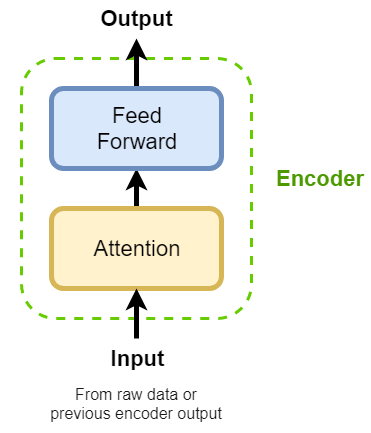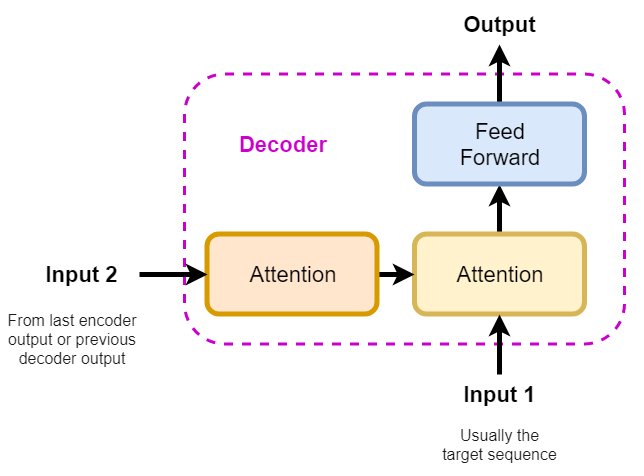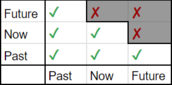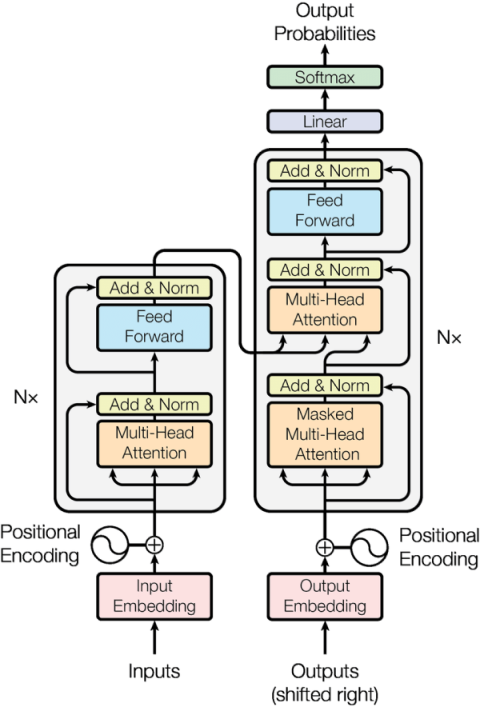# Transformer in 5 minutes

Blue Season · September 8, 2019

The transformer architecture from Attention is all you need is the most important technology for natural language processing in recent years. This post is basically a TL;DR + ELI5 version explaining this ingenious model design.

All code shown here are powered by PyWarm, a high level PyTorch API that makes the network definitions super clean.

## Transformer• Transformer == 6 encoders + 6 decoders

• The encoders encode a source sequence (for example, an English sentence) into a memory representation

• The decoders compare the memory with a target sequence (for example, a corresponding sentence in Spanish) to gain experience in order to handle future situations

``````def transformer(x, y, **kw):
""" x is the source sequence, y is the target sequence """
x = encoder(x, **kw)
x = decoder(x, y, **kw)
return x
``````

## EncoderEach encoder consists of two blocks:

• A self-attention block, that compares different positions in the source sequence to evaluate their relative importances (weights)

• A feed-forward block, that learns the memory representation

• We will discuss these two blocks shortly

``````def encoder(x, num_encoder=6, **kw):
for i in range(num_encoder):
x = residual_add(x, feed_forward, **kw)
return W.layer_norm(x)
``````

## DecoderEach decoder consists of three blocks:

• A self-attention block for the memory (i.e. the output of the encoder stack)

• Because the decoder has access to the entire memory sequence all at once, for any time steps, it may think that future steps can contribute to the past steps, which breaks causality

• To ensure causality, a mask is used to prevent the future leaks into the past:• A memory-target attention block that compares memory with target to establish the relative importances

• A feed-forward block, that learns to gain experience for future tasks

``````def decoder(x, y, num_decoder=6, mask_x=None, mask_y=None, **kw):
""" x is the memory sequence, y is the target sequence """
for i in range(num_decoder):
y = residual_add(y, feed_forward, **kw)
return W.layer_norm(y)
``````

## Feedforward

A feed-forward block is just two linear layers one after another

• A linear layer (sometimes also called dense, or fully connected layer) is essentially the most basic form of neural networks.
``````def feed_forward(x, size_ff=2048, dropout=0.1, **kw):
y = W.linear(x, size_ff, activation='relu')
y = W.dropout(y, dropout)
return W.linear(y, x.shape)
``````

## Attention

The attention mechanism is often considered the most important contribution of the transformer architecture.

• In the most general sense, attention maps one sequence with another sequence to establish relative importance between all pairs of elements

• If the second sequence is the same as the first one, it is then a self-attention

• The attention algorithm works as follows:

• First, a query vector is derived from the first sequence

• Then, a pair of key vector and value vectors are derived from the second sequence

• The similarity between the key and query is measured and used as the attention weights

• The attentions are then applied to the value vector to get a memory vector as output

• A multi-head attention is obtained by splitting the key, query and value into shorter vectors to work in parallel

• This may work better than a single-head attention because each head may attend to different parts better
``````def multi_head_attention(
return t.reshape(batch, -1, t.shape[-1])
if y is None:
y = x # self attention
batch, size = x.shape[:2]
assert size%num_head == 0
assert y.shape[:2] == x.shape[:2]
q = W.linear(x, size) # query
k = W.linear(y, size) # key
v = W.linear(y, size) # value
a = q.transpose(2, 3).contiguous().matmul(k) # attention weights
if mask is not None:
a = F.softmax(a, dim=-1)
a = W.dropout(a, dropout)
x = v.matmul(a.transpose(2, 3).contiguous())
return W.linear(x, size)
``````

## Residual Connection

• All blocks in the transformer use the residual connection paradigm, which enables deeper neural networks

• In each block there is also a normalization step, which makes the network easier to train

``````def residual_add(x, layer, dropout=0.1, **kw):
y = W.layer_norm(x)
y = layer(y, **kw)
y = W.dropout(y, dropout)
return x+y
``````
• Technically, the transformer architecture from the original paper also includes (word) embedding and positional encoding for the source and target, but usually people consider these as preprocessing steps and not part of the transformer. Therefore, we will skip them this time and cover these topics in later posts. Just remember they preprocess the input sequences into a format better suited for neural networks.

• Attention is all you need

• The architecture diagram from the original paper is also included here as a reference